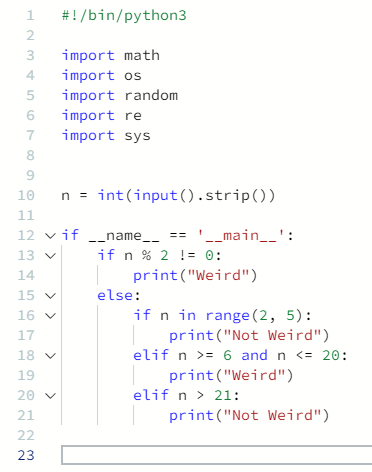# Hackerrank – Python – Introduction – If-else – Solution

Publish Date - November 4th, 2022

|

Hi there! Here’s my solution for the Hackerrank Python introduction for If-else! Ultimately, this an interesting test of basic Python conditionals, while providing a level of difficulty that is around EASY.

Note: In Hackerrank, I moved around the n variable input since if it’s nested in the IF statement, the browser will return no value, thereby being incorrect.

## Problem

Given an integer, , perform the following conditional actions:

If  is odd, print `Weird`

If  is even and in the inclusive range of  2 to 5, print `Not Weird`

If  is even and in the inclusive range of  6 to 20, print `Weird`

If  is even and greater than 20, print `Not Weird`

Input Format

A single line containing a positive integer, .

Output Format

Print `Weird` if the number is weird. Otherwise, print `Not Weird`.

Sample Input 0

``````3
``````

Sample Output 0

``````Weird
``````

Explanation 0

n = 3
n is odd and odd numbers are weird, so print `Weird`.

Sample Input 1

``````24
``````

Sample Output 1

``````Not Weird
``````

Explanation 1

n = 24

n > 20  and n is even, so it is not weird.

## The Solution

Here’s my solution (which should work)

``````#!/bin/python3

import math
import os
import random
import re
import sys

n = int(input().strip())

if __name__ == '__main__':
if n % 2 != 0:
print("Weird")
else:
if n in range(2, 5):
print("Not Weird")
elif n >= 6 and n <= 20:
print("Weird")
elif n > 21:
print("Not Weird")``````

Your output should pass all of the unit tests and sample test cases afterwards when using this code above.

These types of problems are always fun and easy, since it’s just a number of conditionals. Breaking down the problem, you can easily handle each condition within the content as an IF or ELIF statement.

Given an integer, , perform the following conditional actions:

Which means that you have to provide an input and subsequent conditionals so, the already generated:

``````n = int(input().strip()) <- input generator for the UI and a strip() function to ensure no lead or trail spaces

and

if __name__ == '__main__': <- Function declaration.``````

If  is odd, print `Weird`

The start if your conditional. The most effective way is to have python look at n and then use modulus to determine if it’s even or not (if a number is divisible by 2, that means it is even and it will always be zero). If this criteria is satisfied – print(“Weird”).

``````if n % 2 != 0:
print("Weird")``````

If  is even and in the inclusive range of  2 to 5 , print `Not Weird`

If  is even and in the inclusive range of 6  to 20  , print `Weird`

If  is even and greater than 20, print `Not Weird`

Next, you need to provide other statements but ensuring it’s nested after the first condition, since this first condition is essentially a master check. The rest of them are secondary checks. I used a range for the first IF statement after the modulus check then subsequently switched to arithmetic operators after.

``````else:
if n in range(2, 5):
print("Not Weird")
elif n >= 6 and n <= 20:
print("Weird")
elif n > 21:
print("Not Weird")``````

Making sure you nest this second IF statement is crucial. If you don’t do this then your logic won’t have a sense of hierarchy.

Here’s the final output:

## Conclusion

This is a great question to get your bearings on conditionals in Python, and get you comfortable working with an input + a function. Ultimately, it’s simple and Python can get way more complicated!

Feel free to check out my articles on related technologies: